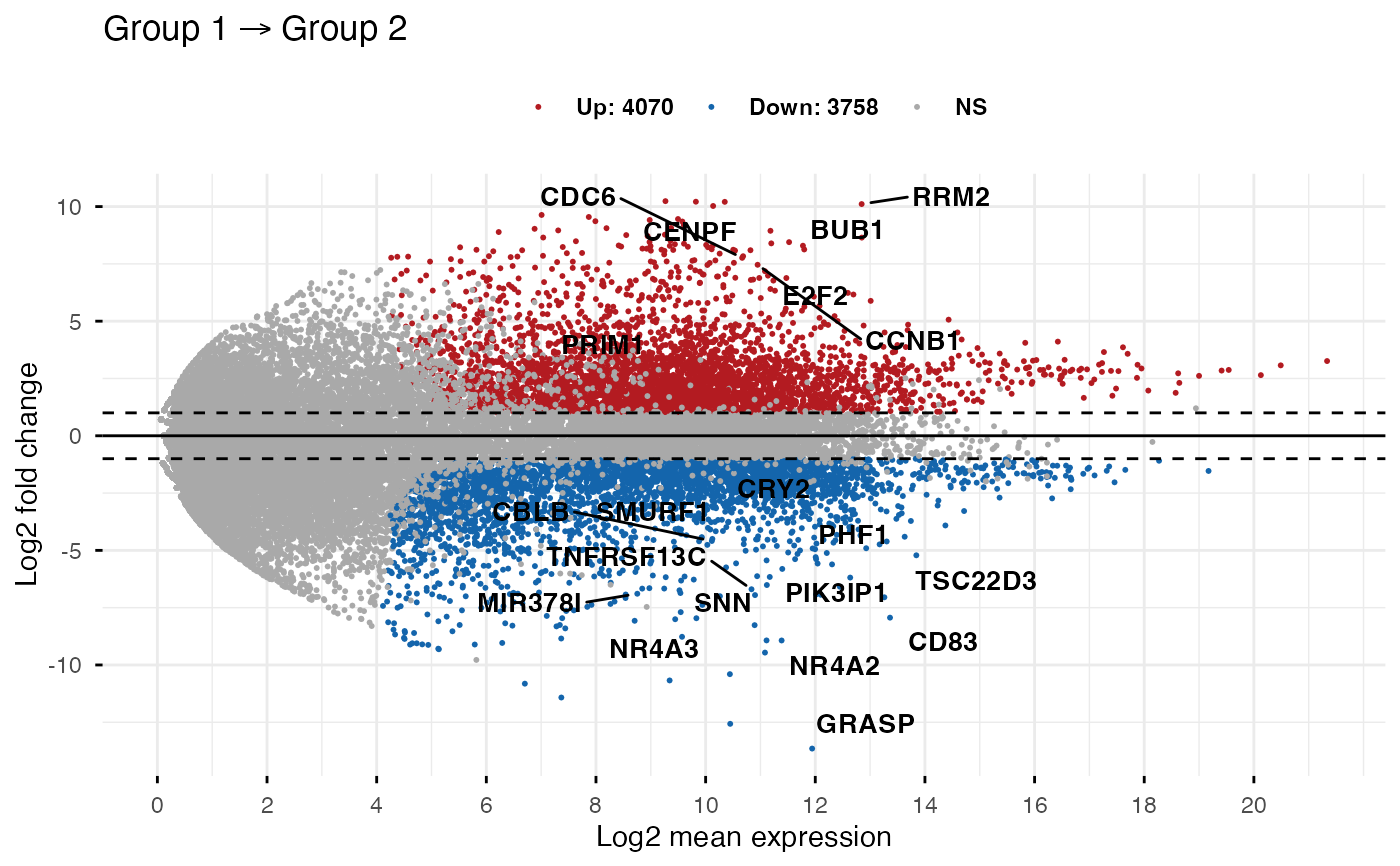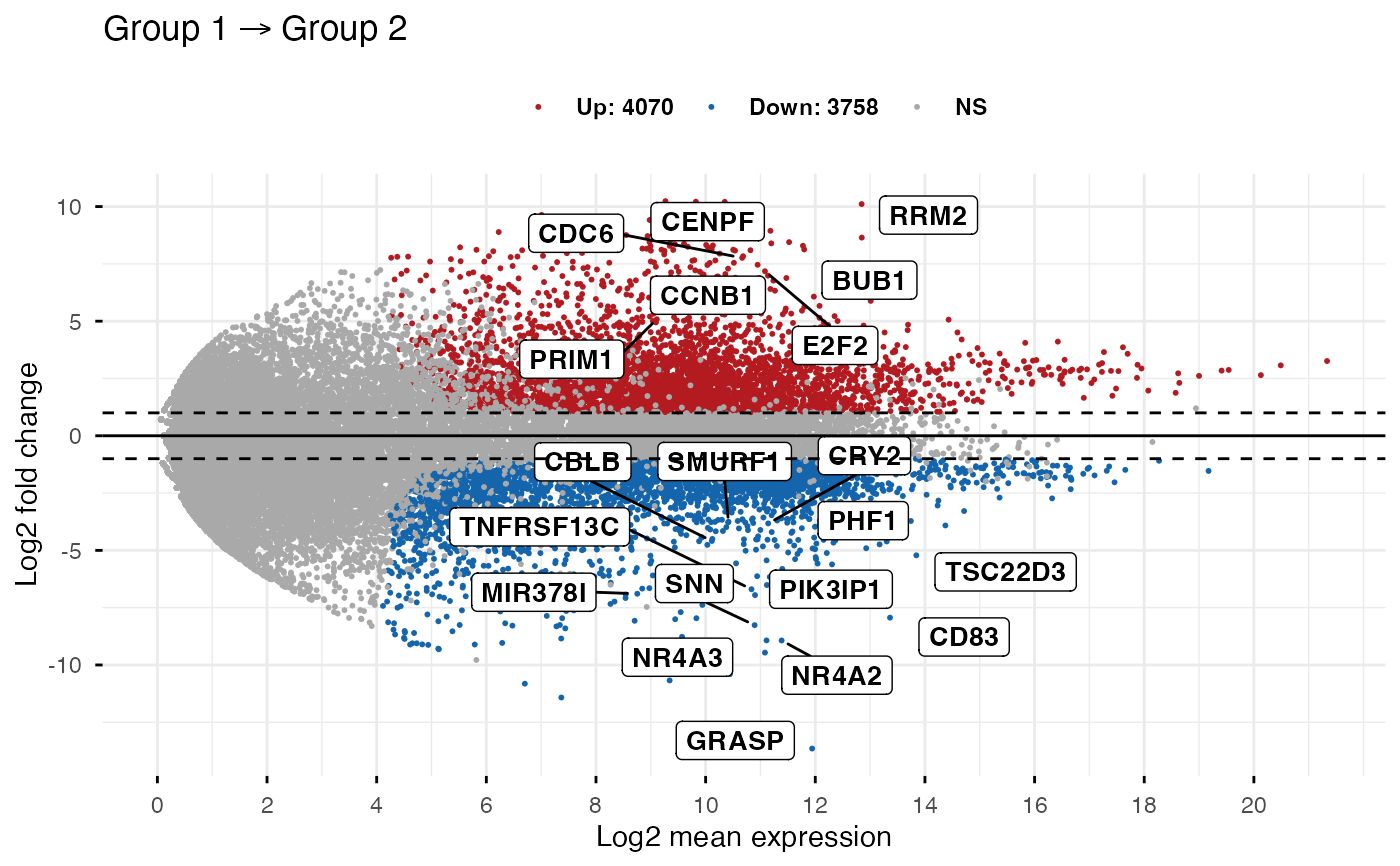Make MA-plot which is a scatter plot of log2 fold changes (M, on the y-axis) versus the average expression signal (A, on the x-axis). M = log2(x/y) and A = (log2(x) + log2(y))/2 = log2(xy)*1/2, where x and y are respectively the mean of the two groups being compared.

ggmaplot(
data,
fdr = 0.05,
fc = 1.5,
genenames = NULL,
detection_call = NULL,
size = NULL,
alpha = 1,
font.label = c(12, "plain", "black"),
label.rectangle = FALSE,
palette = c("#B31B21", "#1465AC", "darkgray"),
top = 15,
label.select = NULL,
main = NULL,
xlab = "Log2 mean expression",
ylab = "Log2 fold change",
ggtheme = theme_classic(),
...
)

## Arguments

data an object of class DESeqResults, get_diff, DE_Results, matrix or data frame containing the columns baseMean (or baseMeanLog2), log2FoldChange, and padj. Rows are genes. Two possible formats are accepted for the input data: 1/ baseMean | log2FoldChange | padj. This is a typical output from DESeq2 pipeline. Here, we'll use log2(baseMean) as the x-axis variable. 2/ baseMeanLog2 | log2FoldChange | padj. Here, baseMeanLog2 is assumed to be the mean of logged values; so we'll use it as the x-axis variable without any transformation. This is the real A in MA plot. In other words, it is the average of two log-scales values: A = (log2(x) + log2(y))/2 = log2(xy)*1/2 Terminology: baseMean: the mean expression of genes in the two groups. log2FoldChange: the log2 fold changes of group 2 compared to group 1 padj: the adjusted p-value of the used statiscal test. Accepted false discovery rate for considering genes as differentially expressed. the fold change threshold. Only genes with a fold change >= fc and padj <= fdr are considered as significantly differentially expressed. a character vector of length nrow(data) specifying gene names corresponding to each row. Used for point labels. a numeric vector with length = nrow(data), specifying if the genes is expressed (value = 1) or not (value = 0). For example detection_call = c(1, 1, 0, 1, 0, 1). Default is NULL. If detection_call column is available in data, it will be used. points size. numeric value betwenn 0 an 1 specifying point alpha for controlling transparency. For example, use alpha = 0.5. a vector of length 3 indicating respectively the size (e.g.: 14), the style (e.g.: "plain", "bold", "italic", "bold.italic") and the color (e.g.: "red") of point labels. For example font.label = c(14, "bold", "red"). logical value. If TRUE, add rectangle underneath the text, making it easier to read. the color palette to be used for coloring or filling by groups. Allowed values include "grey" for grey color palettes; brewer palettes e.g. "RdBu", "Blues", ...; or custom color palette e.g. c("blue", "red"); and scientific journal palettes from ggsci R package, e.g.: "npg", "aaas", "lancet", "jco", "ucscgb", "uchicago", "simpsons" and "rickandmorty". the number of top genes to be shown on the plot. Use top = 0 to hide to gene labels. methods to be used for selecting top genes. Allowed values include "padj" and "fc" for selecting by adjusted p values or fold changes, respectively. character vector specifying some labels to show. plot main title. character vector specifying x axis labels. Use xlab = FALSE to hide xlab. character vector specifying y axis labels. Use ylab = FALSE to hide ylab. function, ggplot2 theme name. Default value is theme_pubr(). Allowed values include ggplot2 official themes: theme_gray(), theme_bw(), theme_minimal(), theme_classic(), theme_void(), .... other arguments to be passed to ggpar.

## Value

returns a ggplot.

## Examples

data(diff_express)

# Default plot
ggmaplot(diff_express, main = expression("Group 1" %->% "Group 2"),
fdr = 0.05, fc = 2, size = 0.4,
palette = c("#B31B21", "#1465AC", "darkgray"),
genenames = as.vector(diff_express$name), legend = "top", top = 20, font.label = c("bold", 11), font.legend = "bold", font.main = "bold", ggtheme = ggplot2::theme_minimal())# Add rectangle around labels ggmaplot(diff_express, main = expression("Group 1" %->% "Group 2"), fdr = 0.05, fc = 2, size = 0.4, palette = c("#B31B21", "#1465AC", "darkgray"), genenames = as.vector(diff_express$name),
legend = "top", top = 20,
font.label = c("bold", 11), label.rectangle = TRUE,
font.legend = "bold",
font.main = "bold",
ggtheme = ggplot2::theme_minimal())# Select specific genes to show
# set top = 0, then specify genes using label.select argument
ggmaplot(diff_express, main = expression("Group 1" %->% "Group 2"),
fdr = 0.05, fc = 2, size = 0.4,
genenames = as.vector(diff_express\$name),
ggtheme = ggplot2::theme_minimal(),
top = 0, label.select = c("BUB1", "CD83")
)